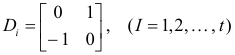# 5 - Matrices and Determinants Questions Answers

Maths >> Algebra >> Matrices and Determinant Engineering Exam

prove using property of determinant

1.  a+b+c      -c                 -b

-c               a+b+c           -a                   =  2(a+b)( b+c ) (c+a)

-b              -a                     a+b+c

Joshi sir comment

first use C1 = C1+C2+C3

then R1 = R1+R2 and R2 = R2+R3

after it solve mathematically

Maths >> Algebra >> Matrices and Determinant Engineering Exam

## which is the 4 digit number whose second digit is thrice the first digit and 3'rd digit is sum of 1'st and 2'nd and last digit is twice the second digit.

Joshi sir comment

let the 4 digit number is 1000a+100b+10c+d

then according to the given condition b=3a, c=a+b, d=2b

so c=4a, d=6a and b=3a

on putting all the values we get number = 1000a+100(3a)+10(4a)+6a = 1346a

on putting a =1, number = 1346

1) Find the invariants of the matrix

0  1  0

0   0  0

0   0  0             of a linear transformation T in A(v) ?

2)   Find the companion matrix of the polynomial ( x+1)2     ?

3)  show that two real symmetric matrices are congruent if and only if they have the same rank and signature?

Joshi sir comment

rank(A) = 1
det(A) = 0
trace(A) = 0
The matrix is not symmetric.

characteristic polynomial of the given matrix is x3

companion matrix of the polynomial (x+1)2

0     1

-1    -2

A real symmetric matrix of rank r is congruent over the field of real numbers to a canonical matrixThe integer p is called the index of the matrix and s = p - (r - p) is called the signature.

The index of a symmetric or Hermitian matrix is the number of positive elements when it is transformed to a diagonal matrix. The signature is the number of positive terms diminished by the number of negative terms and the total number of nonzero terms is the rank.

now solve it

left hand derivative of  f(x) = [x]sin( pie x) at x=k where k is an integer,

Joshi sir comment

Left hand derivative of given function at x = k (k is an integer) is

limh -> 0 {f(k-h) - f(k)}/ {k-h-k}

= lim-> 0 {[k-h] sin π(k-h) - [k] sin πk}/{-h}                  now 2 cases arise for even and odd k

first case

= limh ->0  (k-1) sin (-πh) - 0 / (-h)                          if k is even ,   here we used sin πk = 0 and sin (2π-x) = sin (-x)

= π(k-1)                   here we used sin (-πx)/(-πx) = 1

second case

= limh->0 (k-1) sin (πh) - 0 / (-h)                            if k is odd,    here we used sin πk = 0 and sin (3π-x) = sin x

= -π(k-1)

Solution by Joshi sir

Left hand derivative of given function at x = k (k is an integer) is

limh -> 0 {f(k-h) - f(k)}/ {k-h-k}

= lim-> 0 {[k-h] sin π(k-h) - [k] sin πk}/{-h}                  now 2 cases arise for even and odd k

first case

= limh ->0  (k-1) sin (-πh) - 0 / (-h)                          if k is even ,   here we used sin πk = 0 and sin (2π-x) = sin (-x)

= π(k-1)                   here we used sin (-πx)/(-πx) = 1

second case

= limh->0 (k-1) sin (πh) - 0 / (-h)                            if k is odd,    here we used sin πk = 0 and sin (3π-x) = sin x

= -π(k-1)

so for even and odd k answer will be different.

f(x)  = integraition of ( t2-t+2 )2005( t2-t-2 )2007( t2-t-6 )2009( t2-t-12 )2011( t2-3t+2 )2012  dt, then sum of values of x,  where maxima of (x)    is

Joshi sir comment

I think the right question is

f(x)  = 0( t2-t+2 )2005( t2-t-2 )2007( t2-t-6 )2009( t2-t-12 )2011( t2-3t+2 )2012  dt

then sum of values of x,  where maxima of f(x) will be obtained is

for maxima f '(x) = 0

by using Newton Lebnitz formula we get

f '(x) =  (x2-x+2 )2005( x2-x-2 )2007( x2-x-6 )2009( x2-x-12 )2011( x2-3x+2 )2012

comparing it with 0 and making factors we will get x = -1, 2, 3, -2, 4, -3, 1

now obtain the maxima point then sum these values